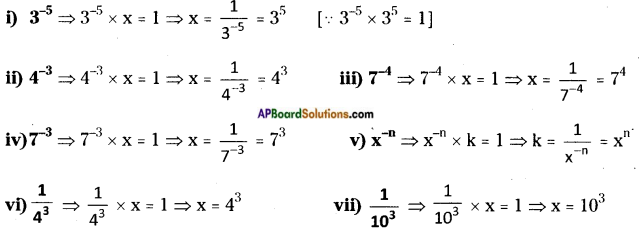## AP State Syllabus 8th Class Maths Solutions 4th Lesson Exponents and Powers InText Questions

AP State Syllabus AP Board 8th Class Maths Solutions Chapter 4 Exponents and Powers InText Questions and Answers.

### 8th Class Maths 4th Lesson Exponents and Powers InText Questions and Answers

Do this

Question 1.
Simplify the following.   (Page No. 81)
i) 37 × 33
ii) 4 × 4 × 4 × 4 × 4
iii) 34 × 43
(i) 37 × 33 = 37 + 33 = 310       [∵ am × an = am+n]
(ii) 4 × 4 × 4 × 4 × 4 = 45      [∵ a × a × a × ……. m times = am]
(iii) 34 × 43 = 34+3 = 37      [∵ am × an = am+n]Question 2.
The distance between Hyderabad and Delhi is 1674.9 km by rail. How would you express this in centimetres? Also express this in the scientific form.     (Page No. 81)
Distance from Hyderabad to Delhi is
= 1674.9 km = 1674.9 × 1000 m = 1674900 mts
= 1674900 × 100 cm
= 167490000 cm
= 16749 × 104 cm

Question 3.
What is 10-10 equal to?     (Page No. 83)
10-10 = $$\frac{1}{10^{10}}$$      [∵ a-n = $$\frac{1}{a^{n}}$$]

Question 4.
Find the multiplicative inverse of the following. (Page No. 83)Question 5.
Expand the following numbers using exponents. (Page No. 84)
i) 543.67
= (5 × 100) + (4 × 10) + (3 × 100) + $$\left(\frac{6}{10}\right)$$ + $$\left(\frac{7}{10^{2}}\right)$$
= (5 × 102) + (4 × 10) + (3 × 100) + (6 × 10-1) + (7 × 10-2)   [∵ an = a-n]

ii) 7054.243
= (7 × 1000) + (0 × 100) + (5 × 10) + (4 × 100) + $$\left(\frac{2}{10}\right)$$ + $$\left(\frac{4}{100}\right)$$ + $$\left(\frac{3}{1000}\right)$$
= (7 × 103) + (0 × 102) + (5 × 101) + (4 × 100) + (2 × 10-1) + (4 × 10-2) + (3 × 10-3)

iii) 6540.305
= (6 × 1000) + (5 × 100) + (4 × 10) + (0 × 100) + $$\left(\frac{3}{10}\right)$$ + $$\left(\frac{0}{100}\right)$$ + $$\left(\frac{5}{1000}\right)$$
= (6 × 103) + (5 × 102) + (4 × 101) + (0 × 100) + (3 × 10-1) + (0 × 10-2) + (5 × 10-3)

iv) 6523.450
= (6 × 1000) + (5 × 100) + (2 × 10) + (3 × 100) + $$\left(\frac{4}{10}\right)$$ + $$\left(\frac{5}{100}\right)$$ + $$\left(\frac{0}{1000}\right)$$
= (6 × 103) + (5 × 102) + (2 × 101) + (3 × 100) + (4 × 10-1) + (5 × 10-2) + (0 × 10-3)

Question 6.
Simplify and express the following as single exponent.    (Page No. 85)
(i) 2-3 × 2-2
(ii) 7-2 × 75
(iii) 34 × 3-5
(iv) 75 × 7-4 × 7-6
(v) m5 × m-10
(vi) (-5)-3 × (-5)-4
(i) 2-3 × 2-2 = 2(-3)+(-2) = 2-5 = $$=\frac{1}{2^{5}}$$ = $$\frac{1}{2 \times 2 \times 2 \times 2 \times 2}$$ = $$\frac{1}{32}$$ [∵ am × an = am+n]
(ii) 7-2 × 75 = 7-2+5 = 73 = 343
(iii) 34 × 3-5 = 34+(-5) = 3-1 = $$\frac{1}{3}$$ [∵ a-n = $$\frac{1}{a^{n}}$$]
(iv) 75 × 7-4 × 7-6 = 75+(-4)+(-6) = 75-10 = 7-5 = $$=\frac{1}{7^{5}}$$
(v) m5 × m-10 = m5+(-10) = m-5 = $$=\frac{1}{m^{5}}$$
(vi) (-5)-3 × (-5)-4 = (-5)(-3)+(-4) = (-5)-7 = $$\frac{1}{(-5)^{7}}$$ = –$$\frac{1}{5^{7}}$$Question 7.
Change the numbers into standard form and rewrite the statements.      (Page No. 93)
i) The distance from the Sun to Earth is 149,600,000,000 m
149,600,000,000 m = 1496 × 108 m

ii) The average radius of the Sun is 695000 km
695000 km = 695 × 103 km

iii) The thickness of human hair is in the range of 0.005 to 0.001 cm.
0.005 to 0.001 cm
= $$\frac{5}{1000}$$ to $$\frac{1}{1000}$$ cm = 5 × 10-3 to 1 × 10-3 cm

iv) The height of Mount Everest is 8848 m
8848 m, itself is a standard form.Question 8.
Write the following numbers in the standard form.      (Page No. 93)
The standard form of the following numbers are
(i) 0.0000456 = $$\frac{456}{10000000}$$ = 456 × 10-7
(ii) 0.000000529 = $$\frac{529}{1000000000}$$ = 529 × 109
(iii) 0.0000000085 = $$\frac{85}{10000000000}$$ = 85 × 1010
(vi) 0.000437 × 104 = $$\frac{437}{1000000}$$ × 104